Courses

# Ideal and Non-ideal Solutions Notes | EduRev

## Class 12 : Ideal and Non-ideal Solutions Notes | EduRev

The document Ideal and Non-ideal Solutions Notes | EduRev is a part of the Class 12 Course Chemistry for JEE.
All you need of Class 12 at this link: Class 12

IDEAL AND NON IDEAL SOLUTIONS
As we know Raoult’s Law states that mole fraction of the solute component is directly proportional to its partial pressure.
On the basis of Raoult’s Law, liquid-liquid solutions are classified into two types of solutions, they are:
•  Ideal Solutions
•  Non-ideal Solutions

Ideal Solutions

• The solutions which obey Raoult’s Law at every range of concentration and at all temperatures are called Ideal Solutions.
• We can obtain ideal solutions by mixing two ideal components that is, solute and a solvent having similar molecular size and structure.
• Example: consider two liquids A and B, and mix them. The formed solution will experience several intermolecular forces of attractions inside it, which will be:
• A – A intermolecular forces of attraction
• B – B intermolecular forces of attraction
• A – B intermolecular forces of attraction
• The solution is said to be an ideal solution, only when the intermolecular forces of attraction between A – A, B – B and A – B are nearly equal.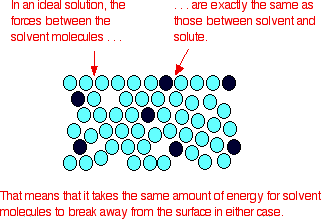Image 2: Molecular structure is same in case of ideal solutions

Ideal Solutions generally have characteristics as follows
• They follow Raoult’s Law, which means partial pressure of components A and B in a solution will be PA = PA0 xA and PB = PB0 xB  where PA0 and PB0 are respective vapour pressure in pure form and xA and xB are respective mole fractions of components A and B
• The enthalpy of mixing of two components should be zero, that is, Δmix H = 0. This signifies that no heat is released or absorbed during mixing of two pure components to form ideal solution
• The volume of mixing of two components should be zero that is, Δmix V = 0. This means that total volume of solution is equal to the sum of the volume of solute and solution. Adding further, it also signifies that there is no occurrence of contraction or expansion of volume while mixing of two components
• The solute-solute interaction and solvent-solvent interaction is nearly equal to solute-solvent interaction

Note: Perfectly ideal solutions are rare in nature, only some solutions show some ideal behavior.

Examples of Ideal Solutions

• n-hexane and n-heptane
• Bromoethane and Chloroethane
• Benzene and Toluene
• CCl4 and SiCl4
• Chlorobenzene and Bromobenzene
• Ethyl Bromide and Ethyl Iodide
• n-Butyl Chloride and n-Butyl Bromide

Non-Ideal Solutions
The solutions which don’t obey Raoult’s law at every range of concentration and at all temperatures are called Non-Ideal Solutions. Non-ideal solutions deviate from ideal solutions and are also known as Non-Ideal Solutions.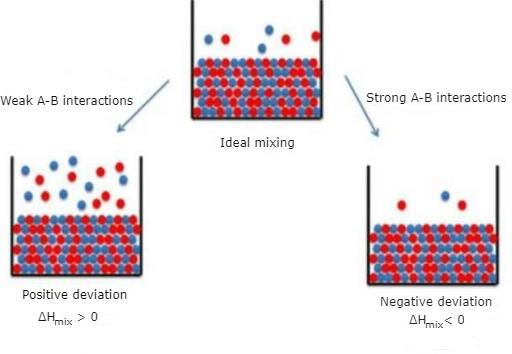Image 1: Types of Non Ideal Solutions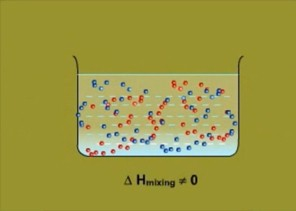Image 4: The enthalpy of mixing is nonzero in non-ideal solution as some heat is released or absorbed in the process.

Non-ideal solutions depict characteristics as follows:

• The solute-solute and solvent-solvent interaction is different from that of solute-solvent interaction
• The enthalpy of mixing that is, Δmix H ≠ 0, which means that heat might have released if enthalpy of mixing is negative  (Δmix H < 0) or the heat might have observed if enthalpy of mixing is positive (Δmix H > 0)
• The volume of mixing that is,  Δmix V ≠ 0, which depicts that there will be some expansion or contraction in dissolution of liquids

Non-ideal solutions are of two types:

• Non-ideal solutions showing positive deviation from Raoult’s Law
• Non-ideal solutions showing negative deviation from Raoult’s Law

Positive Deviation from Raoult’s Law
Positive Deviation from Raoult’s Law occurs when the vapour pressure of component is greater than what is expected in Raoult’s Law.
Example: consider two components A and B to form non-ideal solutions.
Let the vapour pressure, pure vapour pressure and mole fraction of component A be PA , PA0 and xA respectively and that of component B be PB , PB0 and xB respectively.

These liquids will show positive deviation when Raoult’s Law when

• PA > PA0 xA and PB > P0B xB, as the total vapour pressure (PA0 xA + P0B xB) is greater than what it should be according to Raoult’s Law.
• The solute-solvent forces of attraction is weaker than solute-solute and solvent-solvent interaction that is, A – B < A – A or B – B
• The enthalpy of mixing is positive that is, Δmix H > 0 because the heat absorbed to form new molecular interaction is less than the heat released on breaking of original molecular interaction
• The volume of mixing is positive that is, Δmix V > 0 as the volume expands on dissolution of components A and B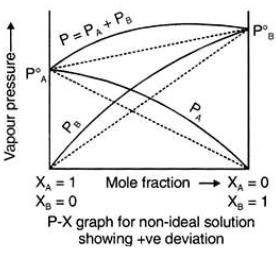Image 3: Graph between vapour
pressure and mole fraction showing
positive deviation

Examples: Following are examples of solutions showing positive deviation from Raoult’s Law

• Acetone and Carbon disulphide
• Acetone and Benzene
• Carbon Tetrachloride and Toluene or Chloroform
• Methyl Alcohol and Water
• Acetone and Ethanol
• Ethanol and Water

NEGATIVE DEVIATION FROM RAOULT’S LAW
Negative Deviation occurs when the total vapour pressure is less than what it should be according to Raoult’s Law. Considering the same A and B components to form a non-ideal solution, it will show negative deviation from Raoult’s Law only when:

• PA < PA0 xA and PB < P0B xB  as the total vapour pressure (PA0 xA + P0B xB) is less than what it should be with respect to Raoult’s Law
• The solute-solvent interaction is stronger than solute-solute and solvent-solvent interaction that is, A – B > A – A or B – B
• The enthalpy of mixing is negative that is, Δmix H < 0 because more heat is released when new molecular interactions are formed
• The volume of mixing is negative that is,  Δmix V < 0 as the volume decreases on dissolution of components A and B

Examples: Following are examples of solutions showing negative deviation from Raoult’s Law

• Chloroform and Benzene
• Chloroform and Diether
• Acetone and Aniline
• Nitric Acid ( HNO3) and water
• Acetic Acid and pyridine
• Hydrochloric Acid ( HCl) and water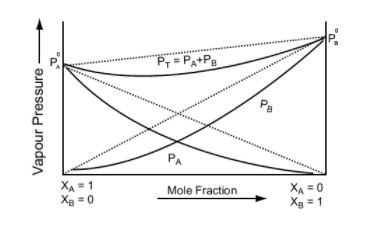Image 5: Graph between vapour pressure and mole fraction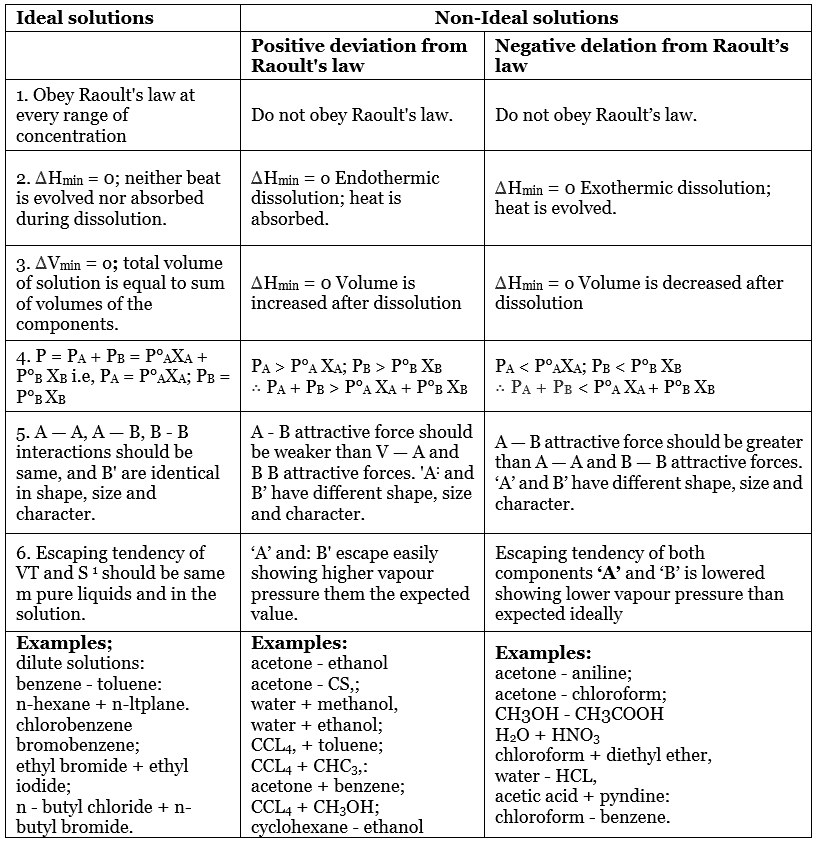Offer running on EduRev: Apply code STAYHOME200 to get INR 200 off on our premium plan EduRev Infinity!

## Chemistry for JEE

223 videos|446 docs|334 tests

,

,

,

,

,

,

,

,

,

,

,

,

,

,

,

,

,

,

,

,

,

;